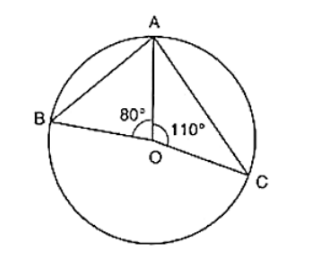# In figure, O is the centre of the circle. Find ∠BAC.Question:

In figure, O is the centre of the circle. Find ∠BAC.Solution:

We have ∠AOB = 80°

And ∠AOC = 110°

Therefore, ∠AOB + ∠AOC + ∠BOC = 360° [Complete angle]

⇒ 80° + 100° + ∠BOC = 360°

⇒ ∠BOC = 360° − 80° − 110°

⇒ ∠BOC = 170°

By degree measure theorem

∠BOC = 2∠BAC

⇒ 170° = 2∠BAC

⇒ ∠BAC = 170°/2 = 85°